Examples

Chapter 13 Class 10 Surface Areas and Volumes (Term 2)
Serial order wise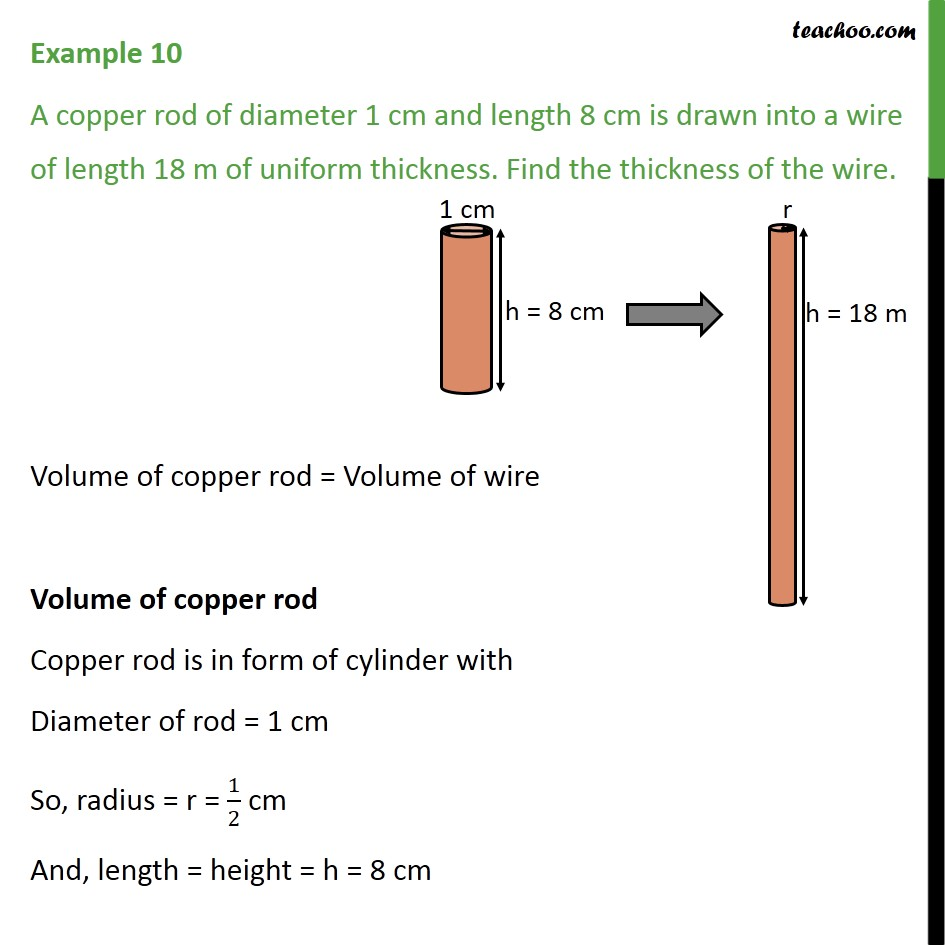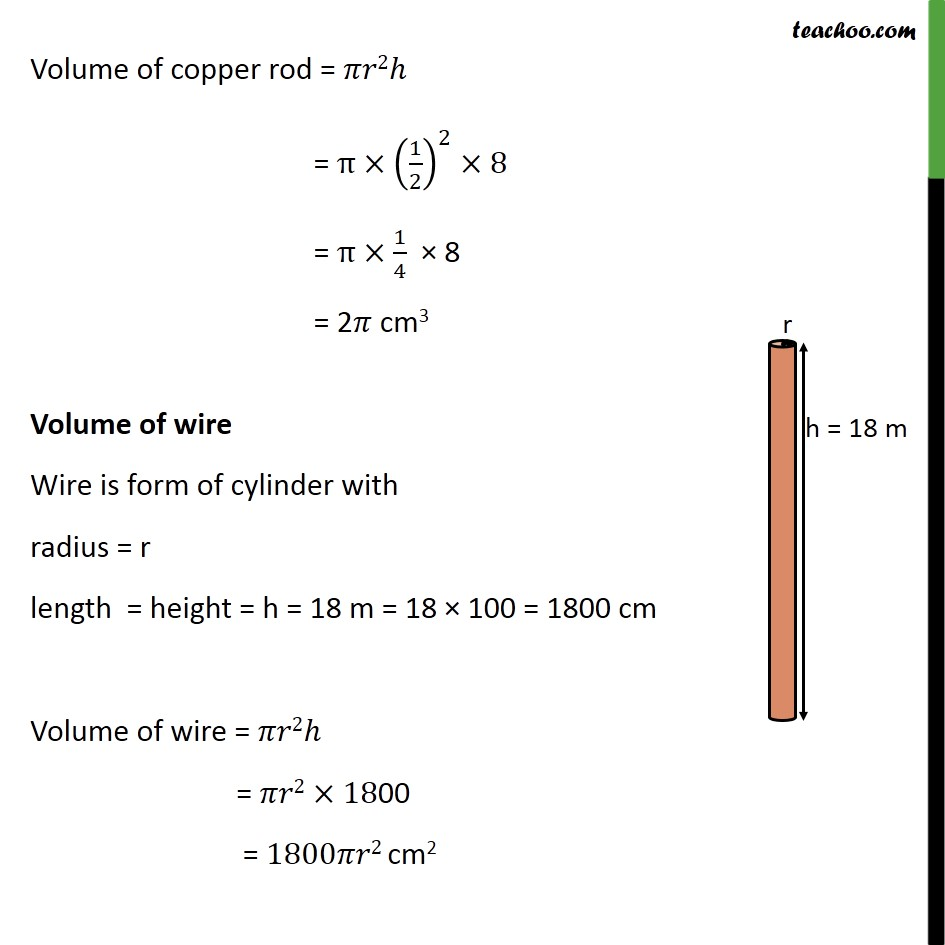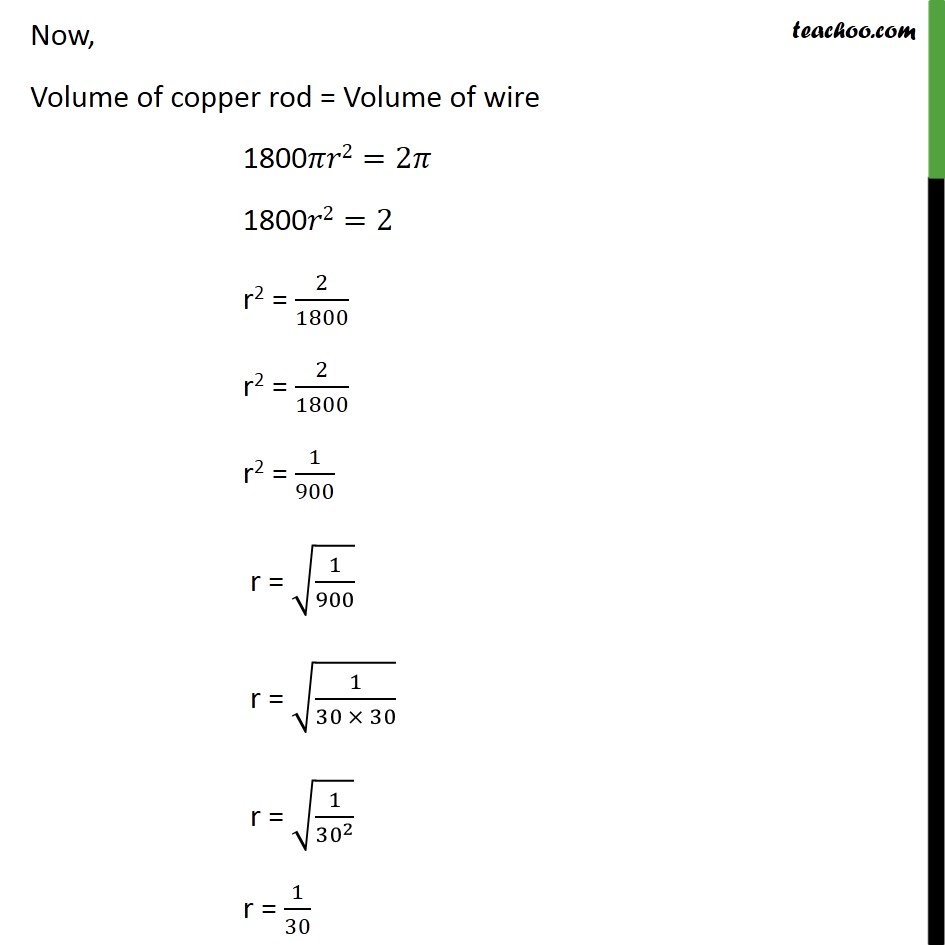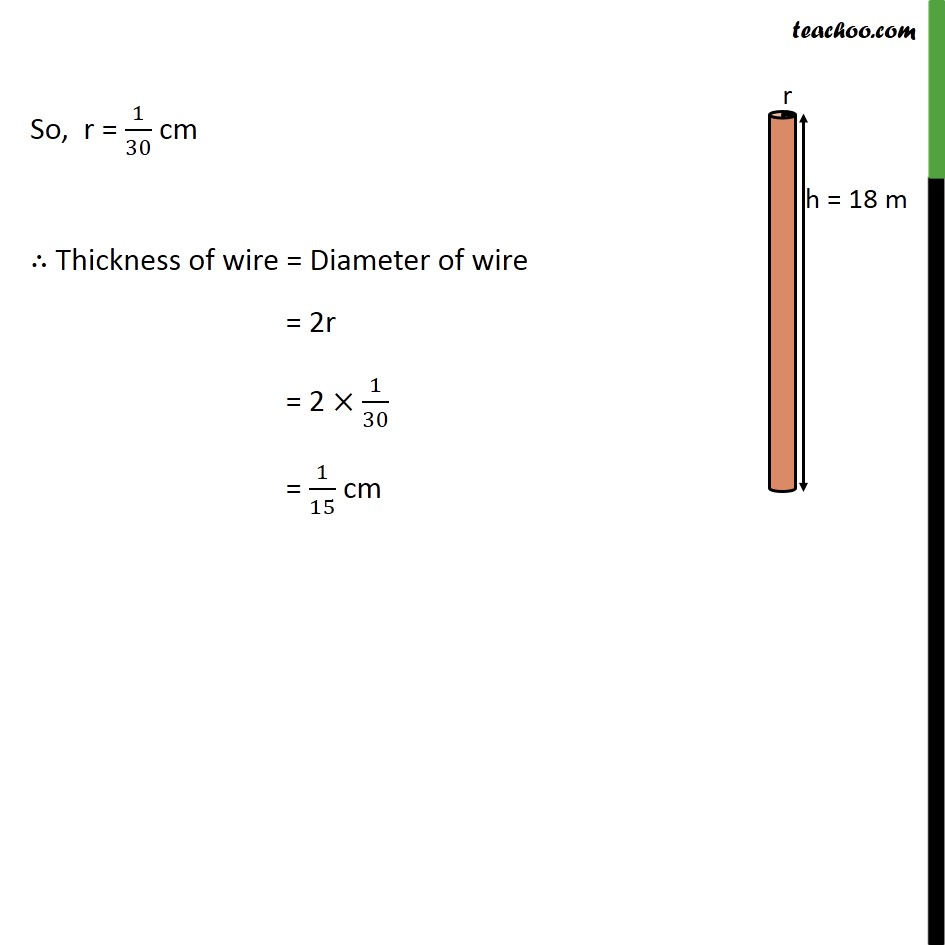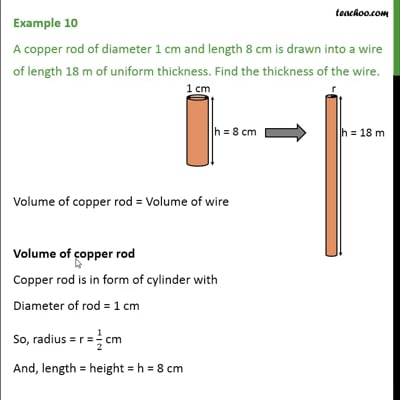This video is only available for Teachoo black users

### Transcript

Example 10 A copper rod of diameter 1 cm and length 8 cm is drawn into a wire of length 18 m of uniform thickness. Find the thickness of the wire. Volume of copper rod = Volume of wire Volume of copper rod Copper rod is in form of cylinder with Diameter of rod = 1 cm So, radius = r = 1/2 cm And, length = height = h = 8 cm Volume of copper rod = 2 = (1/2)^2 8 = 1/4 8 = 2 cm3 Volume of wire Wire is form of cylinder with radius = r length = height = h = 18 m = 18 100 = 1800 cm Volume of wire = 2 = 2 1800 = 1800 2 cm2 Now, Volume of copper rod = Volume of wire 1800 2=2 1800 2=2 r2 = 2/1800 r2 = 2/1800 r2 = 1/900 r = (1/900) r = (1/(30 30)) r = (1/ 30 ^2 ) r = 1/30 So, r = 1/30 cm Thickness of wire = Diameter of wire = 2r = 2 1/30 = 1/15 cm